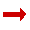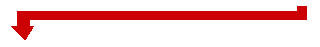# Always End With 1089

### Add two numbers together and always end with 1089. Here is How:

Pick a three digit number. The three digits used must be different*. i.e. 123Reverse that number. 123 becomes 321Take the smallest three digit number from the largest.
321 − 123 = 198Take the answer and reverse that number. 198 becomes 891891 + 198 = 1089### Footnotes

* But I ended with 198 not 1089 ... !

This happens if you pick a number like 546.

546 goes like this: 645−546=99, then 99+99=198.

BUT if you assume that 099 is a 3 digit number, you will get:
990−099=891, and 891+198=1089

* If you don't pick three different digits then this might happen:
343−343=0, 0+0=0